# npm

4.1.1 • Public • Published

The Gamepad API has a standard mapping of axes and buttons. Not all browsers and devices align.

This library is for:

• mapping the button and stick combinations onto common names

This library is not about reading the input from the gamepad controllers. This library is after you've got the input and now you want to make sense of it but you're not sure what controller the player has nor do you know what browser the client is on.

# Usage

`npm i gamepad-api-mappings -S`

## Get the map and deadzone information

```import {getMapping} from 'gamepad-api-mappings';

Deadzones are a way to ignore small input near the centre of the stick (or along an axis). This stops jittery movement when there are no fingers on the stick (human hands are good dampeners).

Included are different ways of factoring deadzones into getting the force. One was is to consider the two axes independently, this results in better snapping along the two axes. Another approach considers the axes as one and this results in a better circular dead zone.

This library has the following algorithms:

• axial (consider each axis independantly)
• radial (consider the two axis together)
• 8 way directional (constrain movement to 4 axes)
• 4 way directional (constrain movement to 2 axes)
• horizontal (constrain movement to the x-axis)
• vertical (constrain movement to y-axis)

```import {axialVector, axial, raw} from 'gamepad-api-mappings';

console.log(force);
console.log(force2.x, force2.y);```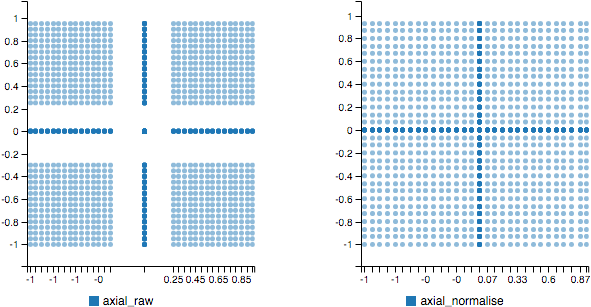```import {radial, raw} from 'gamepad-api-mappings';

console.log(force.x, force.y);```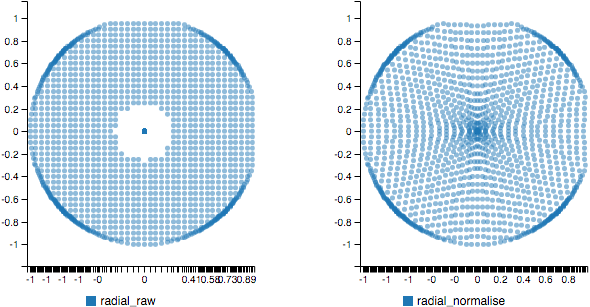### 8 Way Directional

```import {way8, normalise} from 'gamepad-api-mappings';

let force = way8(coord, deadZones['leftStick'], normalise);
console.log(force.x, force.y);```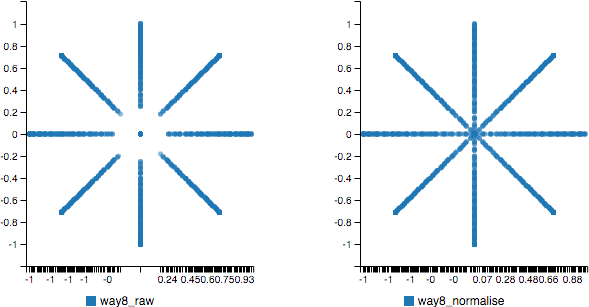### 4 Way Directional

```import {way4, normalise} from 'gamepad-api-mappings';

let force = way4(coord, deadZones['leftStick'], normalise);
console.log(force.x, force.y);```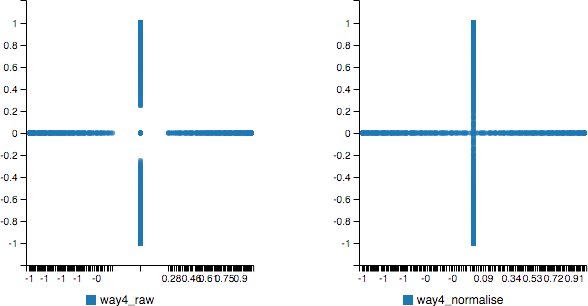### Vertical

```import {vertical, normalise} from 'gamepad-api-mappings';

let force = vertical(coord, deadZones['leftStick'], normalise);
console.log(force.x, force.y);```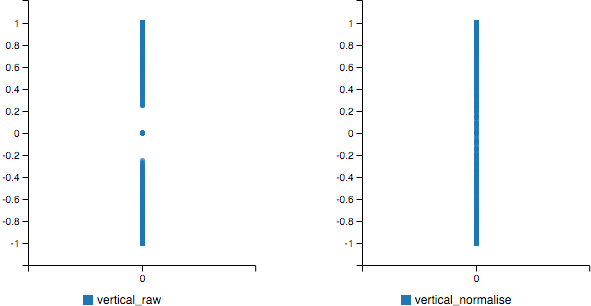### Horizontal

```import {horizontal, normalise} from 'gamepad-api-mappings';

let force = horizontal(coord, deadZones['leftStick'], normalise);
console.log(force.x, force.y);```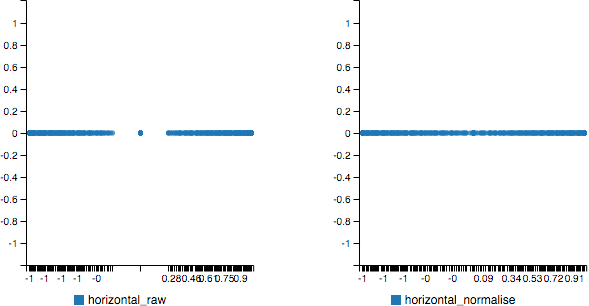## Post processing

The problem with the functions above is that input is zero within the deadzone and then jumps to 0.2 or 0.25 or whatever the size of the dead zone is. The jump is but half of it. With a dead zone of 0.25 we are losing a quarter of the resolution.

This library allows you to pass a function into the algorithms above and adjust the magnitude to suit your game's needs.

We have two mapping algorithms: `raw` and `normalised`. The raw version is in the examples above and does not change the output. Normalisation adjusts the remaining distance so it scales linearly after the dead zone from 0.0 to 1.0. You can supply your own function if it takes a scalar and returns one.

```import {radial, raw, normalise} from 'gamepad-api-mappings';

}

const coord = {x: 0.3, y: 0.9};
console.log(rawPost.x, rawPost.y);                //(0.30, 0.90)
console.log(normalisedPost.x, normalisedPost.y);  //(0.07, 0.86)
console.log(myFuncPost.x, myFuncPost.y);          //(0.08, 0.24)```

An alias for `normalize` exists.

## Keywords

### Install

`npm i @rodes/gamepad-api-mappings`

0

4.1.1